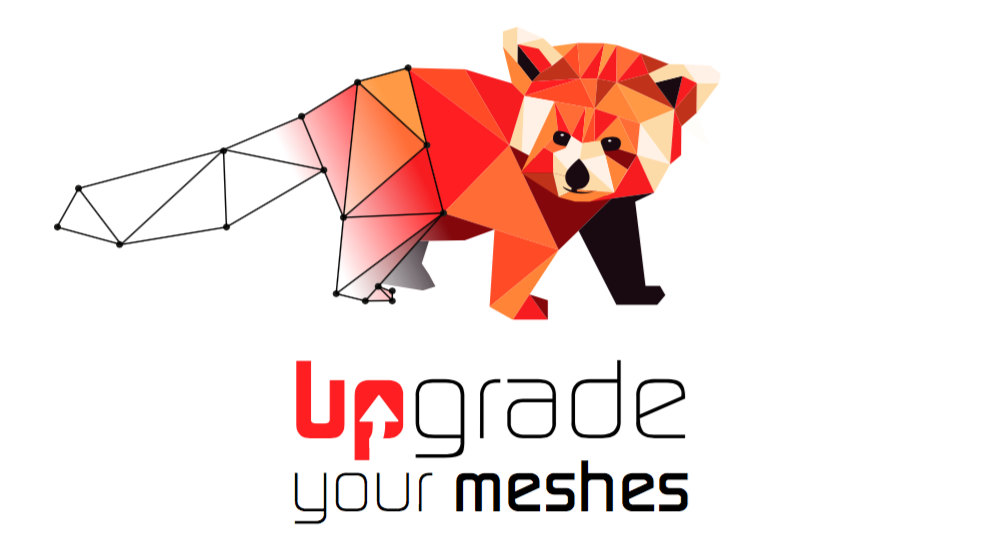# How to scale the metric for anisotropic meshing in MMG2D? Example provided

Hello,
there is only one recently added example of how to set a tensorial metric in MMG2D, for anisotropic meshing but it relies on a mesh/sol file. I thought it would be great to have an example that demonstrates:

• how to first compute a mesh from boundary using MMG2D_mmg2dmesh
• and then how to remesh it using MMG2D_mmg2dlib using an anisotropic metric.

so that the example is self-contained and does not rely on a mesh/sol file, so that one learns from the example how to compute the metric. Since I did not find such an example, I wrote one. The most fundamental example is meshing the coordinate space of a sphere in terms of longitude/latitude, with the objective that the metric makes sure that the distortion disappears when mapped to 3d:

https://gist.github.com/Mathias-Fuchs/b8e9db7a40e304f85f9a481f0a3178fb

and basically, it works, the resulting 3d mesh looks like this:

https://pasteboard.co/J9uM8Kz.png

and the resulting 2d mesh looks like this:

https://pasteboard.co/J9uS1RB.png

which are both fine.
HOWEVER, and this is where I need your help, I don’t know how to scale the metric. In line 52 I have to define a factor of 1000 to pre-multiply the metric with, and this number was just found by trial and error. It did not work just with a factor of one. Empirically, I found that this factor somehow seems to govern the edge size of the resulting mesh (which can’t be set by hsiz), but what exactly is the relationship between that scaling factor of the metric and the resulting edge lengths? How to find that factor in general from the mesh of departure?

I will be happy to submit this example for inclusion in the mmg libexamples folder if it is of interest.
Also, is there another way to achieve an undistorted spherical mesh directly in MMGS instead of MMG2D?
Thanks for any help.

Hello Mathias,

First of all, thank you for this example of usage. I would be happy to integrate it in the library examples: is it ok for you if I fork you example so I can made minor modifications (for example to add ouputs at Medit format (it makes easier the metric visualization) and save the final sphere mesh at Gmsh and Medit format instead of .obj one)?

Regarding the metric scaling: the easier things to do when trying to understand the metric behaviour is to compute the the prescribed sizes and their directions.

Without any factor, you ask for edges of unitary length in the metric M=\left( \begin{array}[cc] \text{cos}(y)^2 & 0 \\ 0 & 1 \end{array} \right).
In the following, I will note (\lambda_1,\lambda_2) the eigenvalues of the matrix and (v_1,v_2) the associated eigenvectors. Here, we simply have:

\left\{ \begin{array}[ccc] \text{} \lambda_1=\text{cos}(y)^2& \text{ associated to }&v_1=(1 \hspace{0.3cm} 0)\\ \text{} \lambda_2 = 1&\text{ associated to }&v_2=(0 \hspace{0.3cm} 1). \end{array} \right.

The wanted edge lengths (s_1,s_2) in the directions of the eigenvalues are related to the matrix eigenvalues (\lambda_1,\lambda_2) such as:

s_i = \frac{1}{\sqrt{\lambda_i}}

Thus, your metric prescribe the size 1 along the y direction and the size \frac{1}{cos(y)} along the x direction (i.e. the size is 1 for y=0 and tends to the infinity for y = ± \pi/2 ). As your sphere mesh has a radius of 1, it is a big size. You can easily fit the size factor f to apply to the s_i values and compute the equivalent factor F = \frac{1}{f^2} to apply to \lambda_i.

To conclude, your initial metric is computed from the tangent vectors \vec{t_x} and \vec{t_y} so it provides a length related to the tangent length.

I hope that it will help you to understand how to compute an anisotropic metric tensor to impose a given size in a given direction.

Regards,
Algiane

Thank you, Algiane!
Ok, this formula for s_i is the missing link. I guess there would have been some plausibility arguments to find it but it’s still good to have it spelled out. Basically, the eigenvalues of the metric indicate the squared speed of the coordinate isolines in the direction of the eigenvectors, so the scaling rule of thumb is “place vertices with density one, and for another density just scale the 3d surface”. Your explanation would greatly benefit the documentation.
Sure, of course you can fork the example. It would just be great if I could submit the example to mmg with a pull request in the end.
Thanks, M

Hi Mathias,

I don’t really know what is the best way to allow you to make a pull request. I don’t know gist but I suppose that it works like git, if yes I can propose:

• to fork your project and to give you access to this fork so you can check my modifications and ask for the PR;
• otherwise, if you give me an access to your gist, I can create a branch, make my modifications on this branch, let you check and ask for the PR.

Just note that for now I don’t know how to give authorization to another user than me to one of my gist fork.

I let you say me if you have a better solution or which one would you perfer.

Regards,
Algiane

Oh I see, one can’t quite collaborate on gists as on usual repos, it seems. So I made a regular repository out of it and invited you as a collaborator:
https://github.com/Mathias-Fuchs/mmg2d_aniso_example
Regards,
Mathias

Hi Mathias,

Thank you. I just push some minor modifications in the new branch feature/for-mmg-integration. I let you review it.

Best,
Algiane